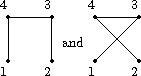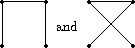Graphs

### Graphs

Example: We would like to derive from Theorem a formula for the number of graphs on v vertices. Before we start doing so it should be mentioned that this example is the second typical example which we present. The first examples were devoted to the introduction of group theoretical concepts, to the derivation of Sylow's Theorem and to a number theoretic result of Fermat. The only example where a suitable choice of GX and Y was made in order to define and enumerate a mathematical structure was in fact the composition of two symmetric groups. As this example may have been a little bit artificial we admit that it is only now that we start to apply our paradigmatic actions more systematically. Let us see how suitably chosen G, X and Y can be used in order to define and enumerate the graphs on v vertices.(Later on we shall refine this method by counting these graphs according to the number of edges or according to their automorphism group. Finally we shall even use this Ansatz in order to construct such graphs and also to generate them uniformly at random.) The way of defining graphs as orbits of groups may at first glance seem to be circumstantial, but we shall see that this definition is very flexible since it can easily be generalized to all other kinds of graphs like multigraphs, directed graphs and so on.
• A labelled (simple) graph consists of a set of vertices and a set of edges joining pairs of vertices, but neither loops (i.e. edges joining a vertex with itself) nor multiple edges are allowed. Thus a labelled graph on v vertices can be considered (after numbering the vertices from 1 to v, say) as a map f from the set [v choose 2] of (unordered) pairs of vertices into the set Y :=2= {0,1 }, where we put
f( {i,j }):= 1 if an edge joins i and j,
and
f( {i,j }):= 0 otherwise.
For example, the first one of the two labelled graphs of figure can be identified in this way with the mapping f :[ 4 choose 2] -> 2 defined by
{1,2 } -> 0, {1,3 } -> 0, {1,4 } -> 1, {2,3 } -> 1, {2,4 } -> 0, {3,4 } -> 1.
• The symmetric group Sv acts on v and hence also on [v choose 2], so that we obtain an action of Sv on 2 [v choose 2] which is of the form G(YX) as was described in the section of paragigmatic examples. Two labelled graphs are called isomorphic if and only if they lie in the same orbit under this action, i.e. if and only if each arises from the other by renumbering the vertices, so that for example the labelled graphs of figure are isomorphic (apply p:=(34) ÎS 4).Two isomorphic labelled graphs with 4 vertices

• A graph   G on v vertices is defined to be such an isomorphism class of labelled graphs. It can be visualized by taking any member of the isomorphism class and deleting the labels.This yields for the labelled graphs shown in figure the drawings of figure.The graphs obtained from the labelled ones above

It should be clear by now what we mean by a graph, and that in our terminology a graph is not a pair (V,E) consisting of a set V of vertices and a set E of edges, but that a graph G can be represented by such a pair, so that, for example, the graphs of figure are in fact equal.
• If we want to allow multiple edges, say up to the multiplicity k, then we again consider X:= [v choose 2], but we change Y into Y:= {0, ...,k }=k+1. The elements of
YX=(k+1) [v choose 2]
are called labelled k-graphs , while by k-graphs on v vertices we mean the orbits of Sv on this set.
• If we want to allow loops or multiple loops, we replace X by the union [v choose 2]È v, and now f(i)=j, for i Îv, means that the vertex with the number i carries a j-fold loop.
• If we want to consider digraphs (i.e. the edges are directed and neither loops nor parallel edges are allowed), we simply put
X := vi2:= {(i,j) Î v2 | i not = j },
the set of injective pairs over v.

Thus graphs, k-graphs, k-graphs with loops and also digraphs can be considered as symmetry classes of mappings. Several other structures will later on be obtained in the same way. Having defined the graphs this way we want to count them by an application of Theorem which means that we have to derive a formula for c( bar ( p)), the number of cyclic factors of bar ( p), the permutation induced by pÎS v on the set [v choose 2] of pairs of points, expressed in terms of the cycle structure of p. In fact we can do better, we can derive the cycle type of bar ( p) from the cycle type of p.

 harald.fripertinger "at" uni-graz.at http://www-ang.kfunigraz.ac.at/~fripert/ UNI-Graz Institut für Mathematik UNI-Bayreuth Lehrstuhl II für Mathematik
last changed: January 19, 2005Graphs